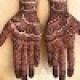# Oscillators Mcqs – (Electrical Engineering) MCQs Latest For FPSC, PPSC, NTS, KPPSC, SPSC & Other Tests

• Home MCQs Oscillators Mcqs – (Electrical Engineering) MCQs Latest For FPSC, PPSC, NTS, KPPSC, SPSC & Other Tests

#### Oscillators Mcqs – (Electrical Engineering) MCQs Latest For FPSC, PPSC, NTS, KPPSC, SPSC & Other Tests

Oscillators Mcqs “. Tab this page to check “Latest Oscillators MCQs” for the preparation of competitive mcqs, FPSC mcqs, PPSC mcqs, SPSC mcqs, KPPSC mcqs, AJKPSC mcqs, BPSC mcqs, NTS mcqs, PTS mcqs, OTS mcqs, Atomic Energy mcqs, Pak Army mcqs, Pak Navy mcqs, CTS mcqs, ETEA mcqs and others. The most occurred mcqs of Oscillators in past papers. Past papers of Oscillators mcqs. Past papers of Oscillators MCQs. Mcqs are the necessary part of any competitive / job related exams. The Oscillators mcqs having specific numbers in any written test. It is therefore everyone have to learn / remember the related Oscillators mcqs. The Important series of Oscillators Mcqs are given below:

An oscillator produces___________oscillations?

A. Modulated
B. Undamped
C. Damped
D. None of the above

If the crystal frequency changes with temperature, we say that crystal has___________temperature coefficient?

A. Positive
B. Negative
C. Zero
D. None of the above

An LC oscillator cannot be used to produce ____________frequencies?

A. Audio
B. High
C. Very low
D. Very high

In an LC transistor oscillator, the active device is___________?

A. Biasing circuit
B. LC tank circuit
C. Transistor
D. None of the above

An oscillator employs___________feedback?

A. Positive
B. Data insufficient
C. Neither positive nor negative
D. Negative

In a phase shift oscillator, we use____________RC sections?

A. Four
B. Three
C. Two
D. None of the above

The application where one would most likely find a crystal oscillator is____________?

A. AF sweep generator
D. None of the above

In a certain oscillator Av = 50. The attention of the feedback circuit must be___________?

A. 01
B. 1
C. 10
D. 02

____________is a fixed frequency oscillator?

A. Hartely-oscillator
B. Phase-shift oscillator
C. Colpitt’s oscillator
D. Crystal oscillator

An important limitation of a crystal oscillator is____________?

A. Its low output
B. Its high output
C. Less availability of quartz crystal
D. Its high Q

The Q of the crystal is of the order of____________?

A. 1000
B. 100
C. 50
D. More than 10,000

In a Wien-bridge oscillator, if the resistances in the positive feedback circuit are decreased, the frequency____________?

A. Remains the same
B. Insufficient data
C. Increases
D. Decreases

A second condition for oscillations is____________?

A. A gain of 1 around the feedback loop
B. The feedback circuit must be capacitive
C. The attention of the feedback circuit must be one-third
D. No gain around the feedback loop

An oscillator differs from an amplifier because it_____________?

A. Requires no d.c. supply
B. Requires no input signal
C. Has more gain
D. Always has the same input

The piezoelectric effect in a crystal is___________?

A. A voltage developed because of mechanical stress
B. A change in frequency because of temperature
C. A change in resistance because of temperature
D. None of the above

In an LC circuit, when the capacitor is maximum, the inductor energy is____________?

A. Minimum
B. Half-way between maximum and minimum
C. Maximum
D. None of the above

A Wien bridge oscillator uses___________feedback?

A. Only negative
B. Only positive
C. Both positive and negative
D. None of the above

Quartz crystal is most commonly used in crystal oscillators because:____________?

A. It has superior electrical properties
B. It is quite inexpensive
C. It is easily available
D. None of the above

The signal generator generally used in the laboratories is___________oscillator?

A. Wien-bridge
B. Crystal
C. Hartely
D. Phase shift

In an LC oscillator, if the value of L is increased four times, the frequency of oscillations is __________?

A. Decreased 4 times
B. Increased 2 times
C. Increased 4 times
D. Decreased 2 times

In Colpitt’s oscillator, feedback is obtained___________?

A. By a tickler coil
B. By magnetic induction
C. From the centre of split capacitors
D. None of the above

For an oscillator to properly start, the gain around the feedback loop must initially be_________?

A. Less than 1
B. Greater than 1
C. 1
D. Equal to attenuation of feedback circuit

One condition for oscillation is_____________?

A. A gain around the feedback loop of one-third
B. A phase shift around the feedback loop of 180o
C. A phase shift around the feedback loop of 0o
D. A gain around the feedback loop of less than 1

The crystal oscillator frequency is very stable due to___________of the crystal?

A. Vibrations
B. Rigidity
C. Low Q
D. High Q

In a phase shift oscillator, the frequency determining elements are _______________?

A. R, L and C
B. L and C
C. R and C
D. None of the above

Hartley oscillator is commonly used in______________?

D. None of the above

In an LC oscillator, the frequency of oscillator is____________L or C?

A. Directly proportional to
B. Proportional to square of
C. Independent of the values of
D. Inversely proportional to square root of

An oscillator converts __________?

A. mechanical power into a.c. power
B. power into a.c. power
C. power into d.c. power
S. none of the above

## 2 Replies to “Oscillators Mcqs – (Electrical Engineering) MCQs Latest For FPSC, PPSC, NTS, KPPSC, SPSC & Other Tests”

1.Aadiya Yousaf says:

hi

1.Muhammad Abdullah says:

W.Salam.

error: